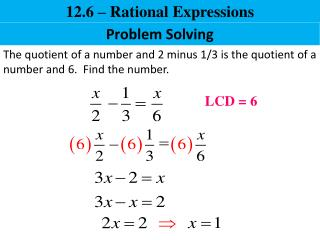# Problem Solving - PowerPoint PPT PresentationDownload PresentationProblem Solving

Problem SolvingDownload Presentation## Problem Solving

- - - - - - - - - - - - - - - - - - - - - - - - - - - E N D - - - - - - - - - - - - - - - - - - - - - - - - - - -
##### Presentation Transcript

1. 12.6 – Rational Expressions Problem Solving The quotient of a number and 2 minus 1/3 is the quotient of a number and 6. Find the number. LCD = 6

2. 12.6 – Rational Expressions Problem Solving If one more than three times a number is divided by the number, the result is four thirds. Find the number. LCD = 3x

3. 12.6 – Rational Expressions Time to sort one batch (hours) Fraction of the job completed in one hour Ryan Mike Together Problem Solving Mike and Ryan work at a recycling plant. Ryan can sort a batch of recyclables in 2 hours and Mike can short a batch in 3 hours. If they work together, how fast can they sort one batch? 2 3 x

4. 12.6 – Rational Expressions Time to sort one batch (hours) Fraction of the job completed in one hour Ryan Mike Together Problem Solving 2 3 x LCD = 6x hrs.

5. 12.6 – Rational Expressions Time to Assemble one unit (hours) Fraction of the job completed in one hour Mary Jim Together Problem Solving Jim and Mary assemble Iron Man action figures. It takes Mary 2 hours to assemble one figure while it takes Jim 8 hours. How long will it take them to assemble one figure if they work together? 2 8 x

6. 12.6 – Rational Expressions Time to Assemble one unit (hours) Fraction of the job completed in one hour Mary Jim Together Problems about Numbers 2 8 x LCD: 8x hrs.

7. 12.6 – Rational Expressions Time to fill a tank (minutes) Fraction of the job completed in one minute 1st pump 2nd pump Together Problem Solving A pump can fill a tank three times as fast as another pump. If the pumps work together, they could fill the tank in fifteen minutes. How long would it take each pump to fill the tank alone? x 3x 15

8. 12.6 – Rational Expressions Time to fill a tank (minutes) Fraction of the job completed in one minute 1st pump 2nd pump Together Problem Solving x 3x 15

9. 12.6 – Rational Expressions Problem Solving 15x LCD: min. min.

10. 12.6 – Rational Expressions Time to pump one basement (hours) Fraction of the job completed in one hour 1st pump 2nd pump Together Problem Solving A sump pump can pump water out of a basement in twelve hours. If a second pump is added, the job would only take six and two-thirds hours. How long would it take the second pump to do the job alone? 12 x

11. 12.6 – Rational Expressions Time to pump one basement (hours) Fraction of the job completed in one hour 1st pump 2nd pump Together Problem Solving 12 x

12. 12.6 – Rational Expressions Problem Solving LCD: 60x hrs.

13. 12.6 – Rational Expressions Problem Solving A boat can travel 9 miles upstream in the same amount of time it takes to travel 11 downstream. If the current of the river is 3 miles per hour, find the speed of the boat going upstream and downstream. distance = rate x time d = r * t d/t = r Rate upstream is 3 mph less than the rate downstream. rupstream = 9/t rdownstream = 11/t LCD = t